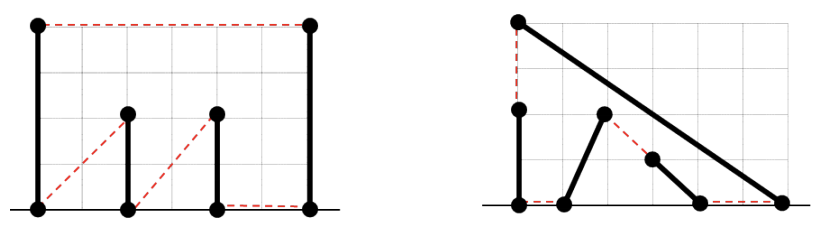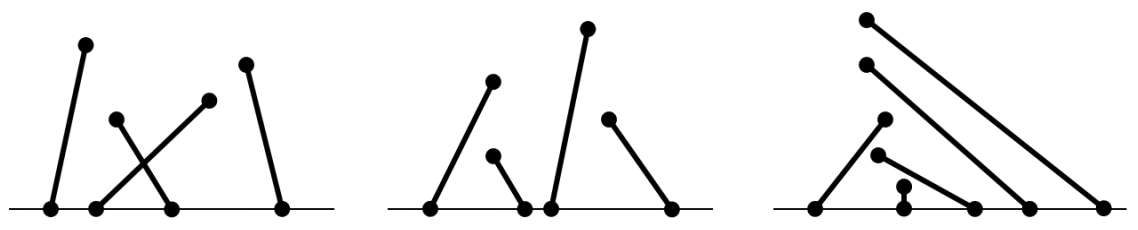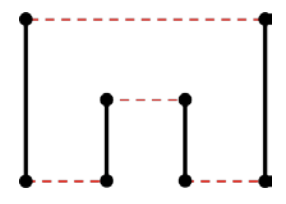시간 제한메모리 제한제출정답맞힌 사람정답 비율
2 초 512 MB6012713.208%

## 문제

Let S be a set of n line segments. An endpoint of every line segment of S lies on the x-axis and the other lies above the x-axis in the plane. All endpoints are distinct, but the segments may intersect at their interiors. We want to draw a simple polygon P such that every line segment of S would be a part of the boundary of P, i.e., either an edge or a part of an edge of P. A polygon P is said to be simple if the angle at each vertex of P is not 180°, and no two edges meet except for the case where two adjacent edges meet at a vertex.

For example, Figure H.1 shows examples of line segments for which simple polygons can be drawn. In Figure, a black solid line segment represents a line segment in a given set S. When combined with red dotted line segments, a simple polygon is constructed. The left one in this figure is a simple poygon such that all endpoints of S become vertices of P. The right one shows a simple polygon only with 6 vertices; two endpoints are each contained in the interior of two edges of P. Note here that an endpoint of a line segment of S does not necessarily have to be a vertex of a simple polygon drawn for S.Figure H.1: Examples of line segments for which simple polygons can be drawn.

On the other hand, Figure H.2 shows examples of line segments for which any simple polygons cannot be drawn.Figure H.2: Examples of line segments for which any simple polygons cannot be drawn.

There may be more than one simple polygon for a set of line segments. In that case, we want to find a simple polygon with the minimum boundary length. For example, the boundary length of the simple polygon shown in Figure H.3 is smaller than that of any other simple polygons for this set.Figure H.3: A simple polygon with the minimum boundary length.

Given a set of line segments, write a program to determine whether there is a simple polygon for the set and if so, to find a simple polygon with the minimum boundary length.

## 입력

Your program is to read from standard input. The input starts with a line containing an integer, n (3 ≤ n ≤ 20,000), where n is the number of line segments. In the following n lines, each line contains three integers x1, x2, and y2 (1 ≤ x1, x2, y2 ≤ 106) which represent the coordinates of the endpoints of a line segment, (x1, 0) and (x2, y2). Note that all endpoints of line segments are distinct, i.e., any two endpoints don’t lie at the same position.

## 출력

Your program is to write to standard output. Print exactly one line. The line should contain a real number rounded to 1 decimal place which represents the boundary length of a simple polygon with the minimum boundary length if there is a simple polygon for a given set of line segments, otherwise, -1.

## 예제 입력 1

4
1 1 2
2 2 1
3 3 1
4 4 2


## 예제 출력 1

12.0


## 예제 입력 2

4
1 1 2
2 3 2
5 4 1
7 1 4


## 예제 출력 2

19.3


## 예제 입력 3

4
5 6 6
4 3 2
1 3 4
9 7 3


## 예제 출력 3

-1